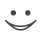This flashcard is just one of a free flashcard set. See all flashcards!

96
A semi-annual pay interest rate swap where the fixed rate is 5.00% (with semi-annual compounding) has a remaining life of nine months.  The six-month LIBOR rate observed three months ago was 4.85% with semi-annual compounding. Today’s three and nine month LIBOR rates are 5.3% and 5.8% (continuously compounded) respectively. From this it can be calculated that the forward LIBOR rate for the period between three- and nine-months is 6.14% with semi-annual compounding.  If the swap has a principal value of \$15,000,000, what is the value of the swap to the party receiving a fixed rate of interest?
A.\$74,250
B.−\$70,760
C.−\$11,250
D.\$103,790